# 📉 PolyPlot

## User can enter any polynomial function and minimum value of x and maximum value of x.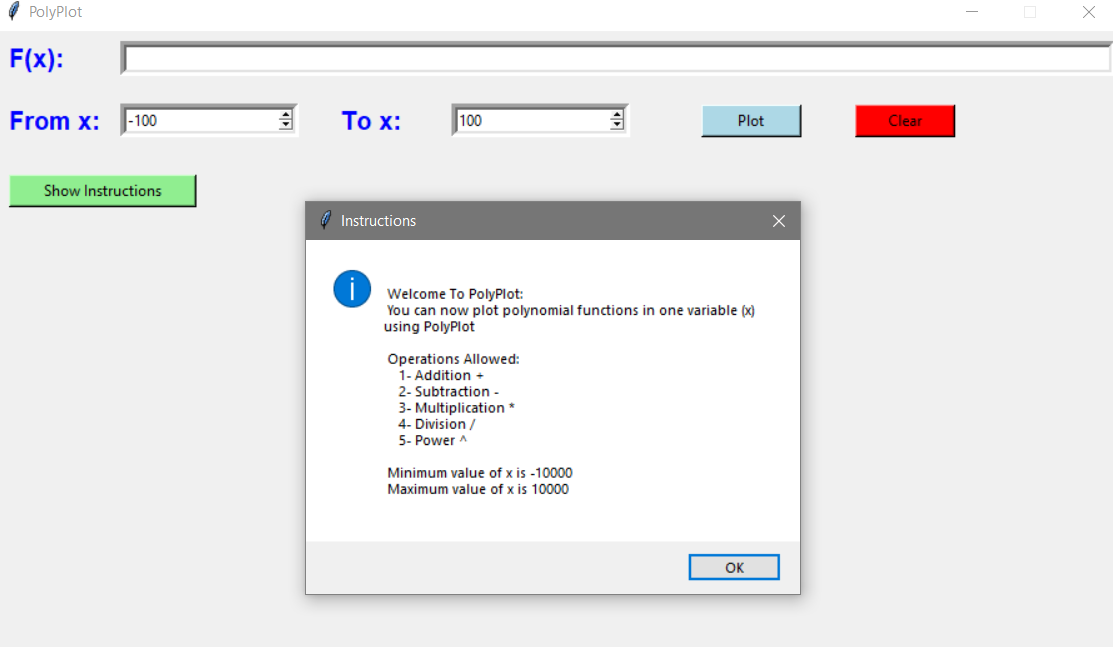# How to use:

## Environment setup:

1. Install Python (+3.6.0).
2. Run the following commands:
• ` pip install numpy`
• ` pip install matplotlib`
• ` pip install tk`
3. Finally, run ` python .\PolyPlot.py`

# How to test:

Run the following command `python .\PolyPlot.py --test true`

# Examples:

### F(x) = x + 3 when x ranges from `0` to `5`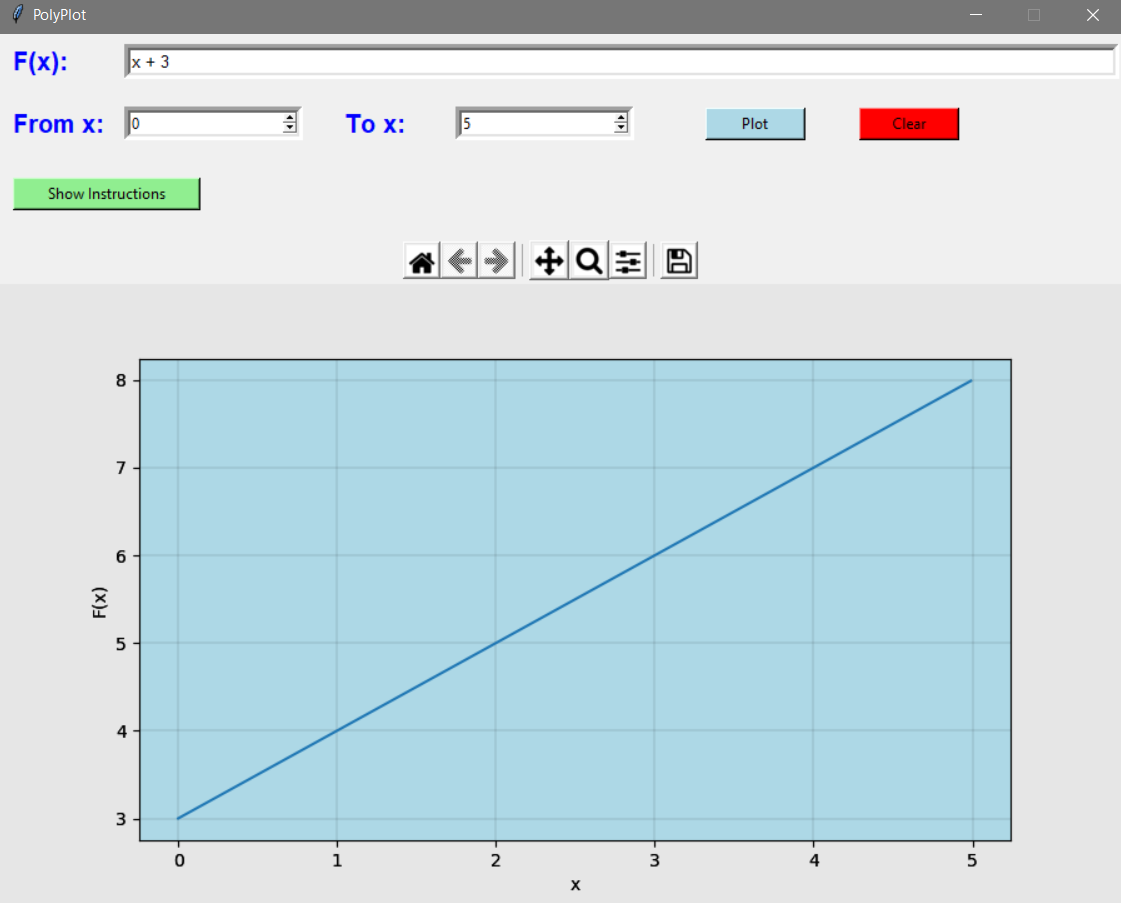### F(x) = x2 + x when x ranges from `100` to `10000`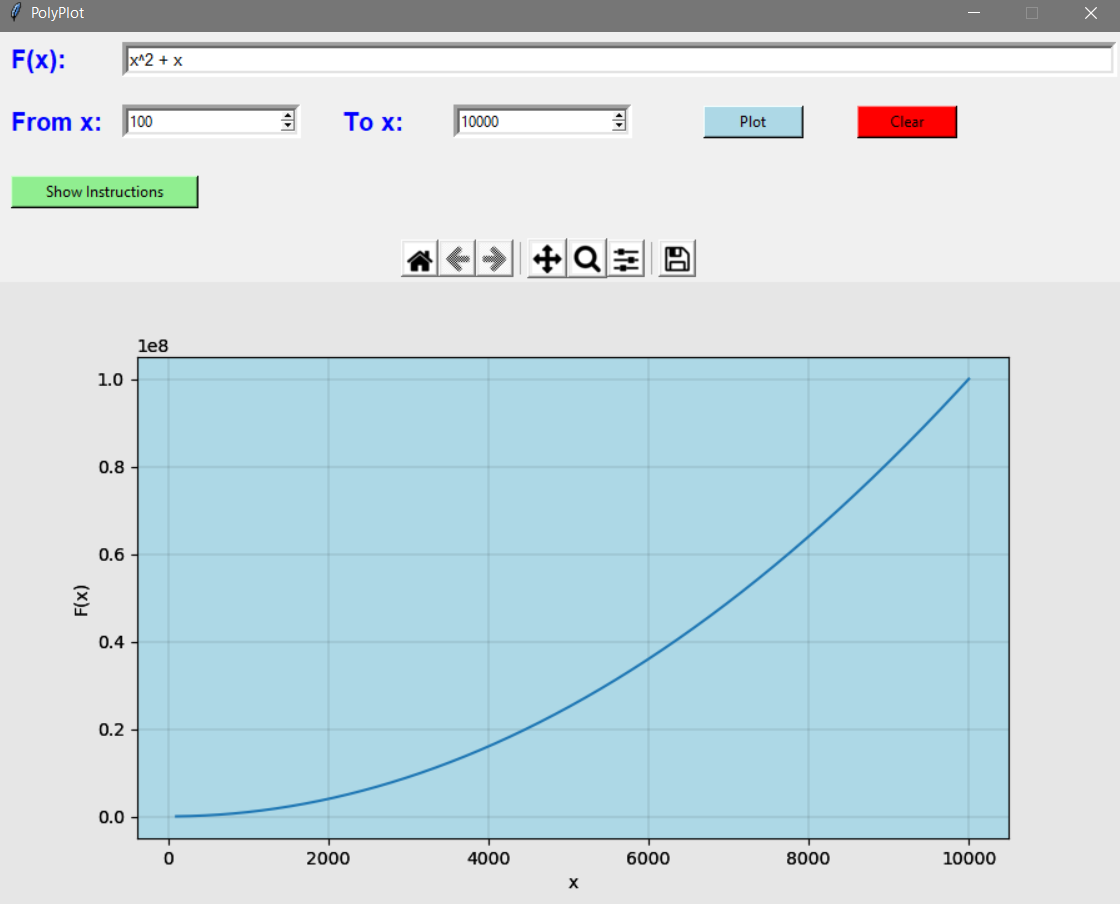### F(x) = 15x3 – 7x2 + x -114 when x ranges from `-200` to `200`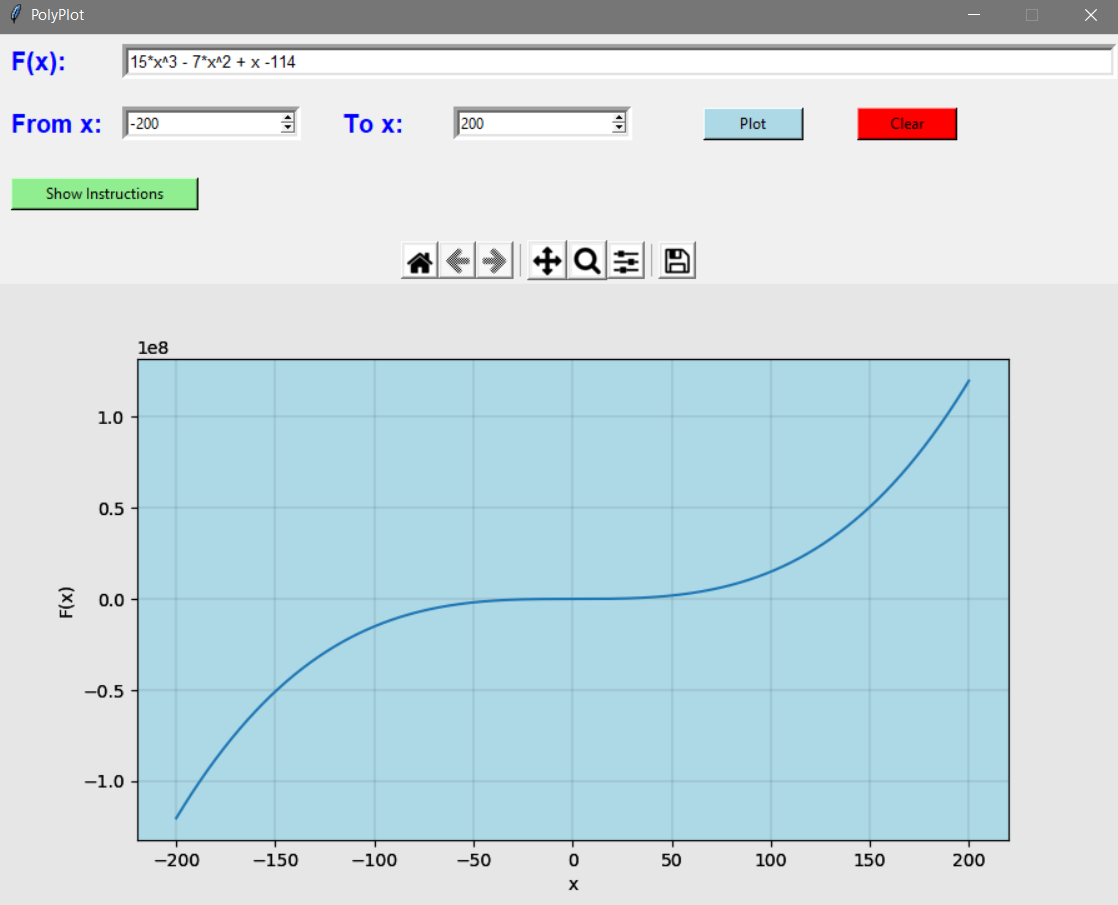# Tests: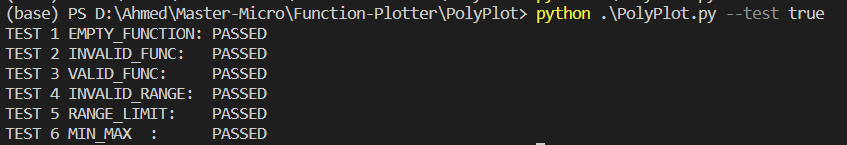View Github